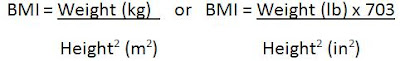Monday, October 26, 2009

Body Mass Index Calculator

The Body Mass Index Calculator or BMI Calculator can be used to determine your level of weight fitness.

The Body Mass Index formula is:The BMI result is categorized as

• Severely Underweight if it is less than 16.4
• Underweight if it is from 16.5 to 18.4
• Normal if it is from 18.5 to 24.9
• Overweight if it is from 25 to 29.9
• Obese Class I if it is from 30 to 34.9
• Obese Class II if it is from 35 to 39.9 and
• Obese Class III if it is 40 and over.

Charles